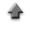home

# Appendices

## 1. Character strings in a graph

• You can display a label of x, y and z axis, and an explanatory note (legend) in a graph.

## 2. Preservation of a gragh image

### Windows (using Paint)

• When you select in order of "Edit"->"Copy" in the menu bar of the graph display, the graph image can be copied onto the clipboard.
• Next, when starting the Paint and selecting from the menu bar of the Paint in order of "Edit(E)"->"Putting (P)", the image is put on the Paint.
• The graph image can be preserved by a suitable file format (jpeg and png, etc.) when you select from the menu bar of the Paint in order of "File (F)"->"Preserve it giving a name (A)".

### Macintosh (using Preview)

• When you select in order of "Edit"->"Copy" in the menu bar of the graph display, the graph image can be copied onto the clipboard.
• Next, when starting the Preview and selecting from the menu bar of the Preview in order of "File"->"New make from the clipboard", the image is put on the Preview.
• The graph image can be preserved by a suitable file format (jpeg and png, etc.) when you select from the menu bar of the Preview in order of "File"->"Preserve it by the alias".

### Linux, Windows and Macintosh (using OpenOffice)

• When you select in order of "Edit"->"Copy" in the menu bar of the graph display, the graph image can be copied onto the clipboard.
• Next, start the OpenOffice, and select graphic depiction (B).
• Select from the menu bar in order of "Edit"->"Putting (P)", and put the image.
• The graph image can be preserved by a suitable file format (png and eps, etc.) when you select from the menu bar in order of "File"->"Export (T)".

## 3. Function model fitting

### Fit summary

• The "correction norm" is the value of the norm of the correction vector. It is the value of the norm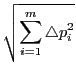, where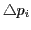(i=1,2,...,m) is the elements of the correction vector which corrects the parameter pi (i=1,2,...,m).
• The "damping factor" is the value of the additional factor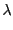of the diagonal elements in the curvature matrix in the normal equation in (Levenberg-)Marquardt method. The value of theis desirable to be 0. If the value of theis not 0, adjust the initial values of the parameters and try to fit again.
• The "probability" shows the probability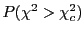in the chi-square distribution that the value of the chi-square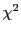exceeds the observed chi-square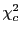for a correct model.
• The residual is defined as the difference between the observed value yi and the function value f(xi) corresponding to x value for the observed value,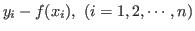. The sum of the suqared residuals(SSR) is also defined as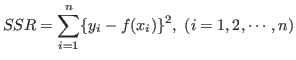.
• The "root mean square(RMS)" is defined as the square root of the sum of the squared residuals(SSR) divided by the number of degrees of freedom(ndf),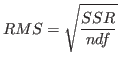. This is a measure of the standard deviation of the residuals.

## 4. Multiple linear regression

The multiple linear regression model assumes that the statistical relationship between the response variable Yi and the explanatory variables xij is of the form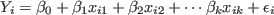,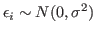,
where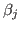(j=0,1,2,...,k) are the regression parameters, xij(i=1,2,...,n, j=1,2,...,k) the explanatory variables,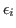denotes the independent normal random variables with zero mean and common variance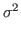.